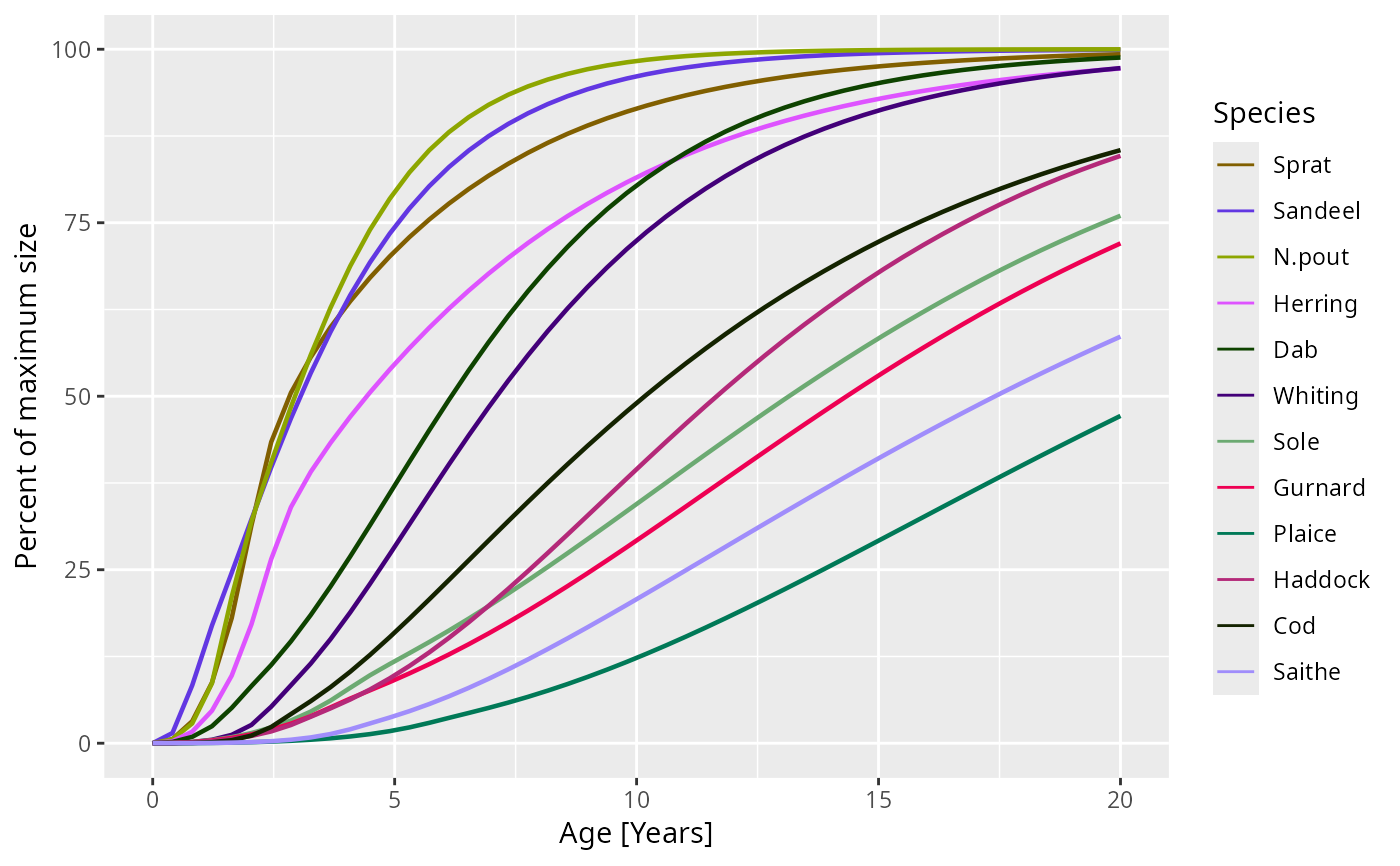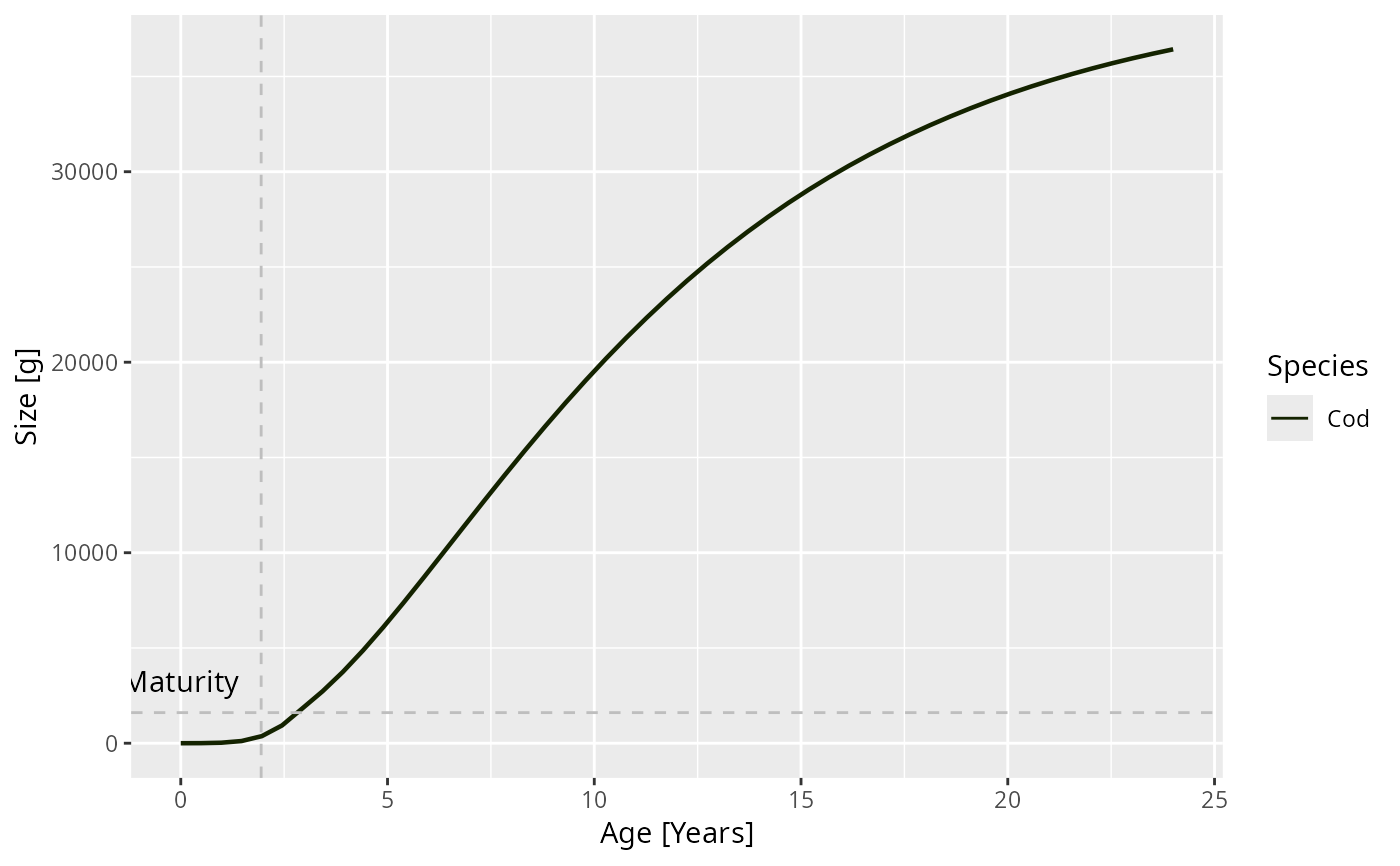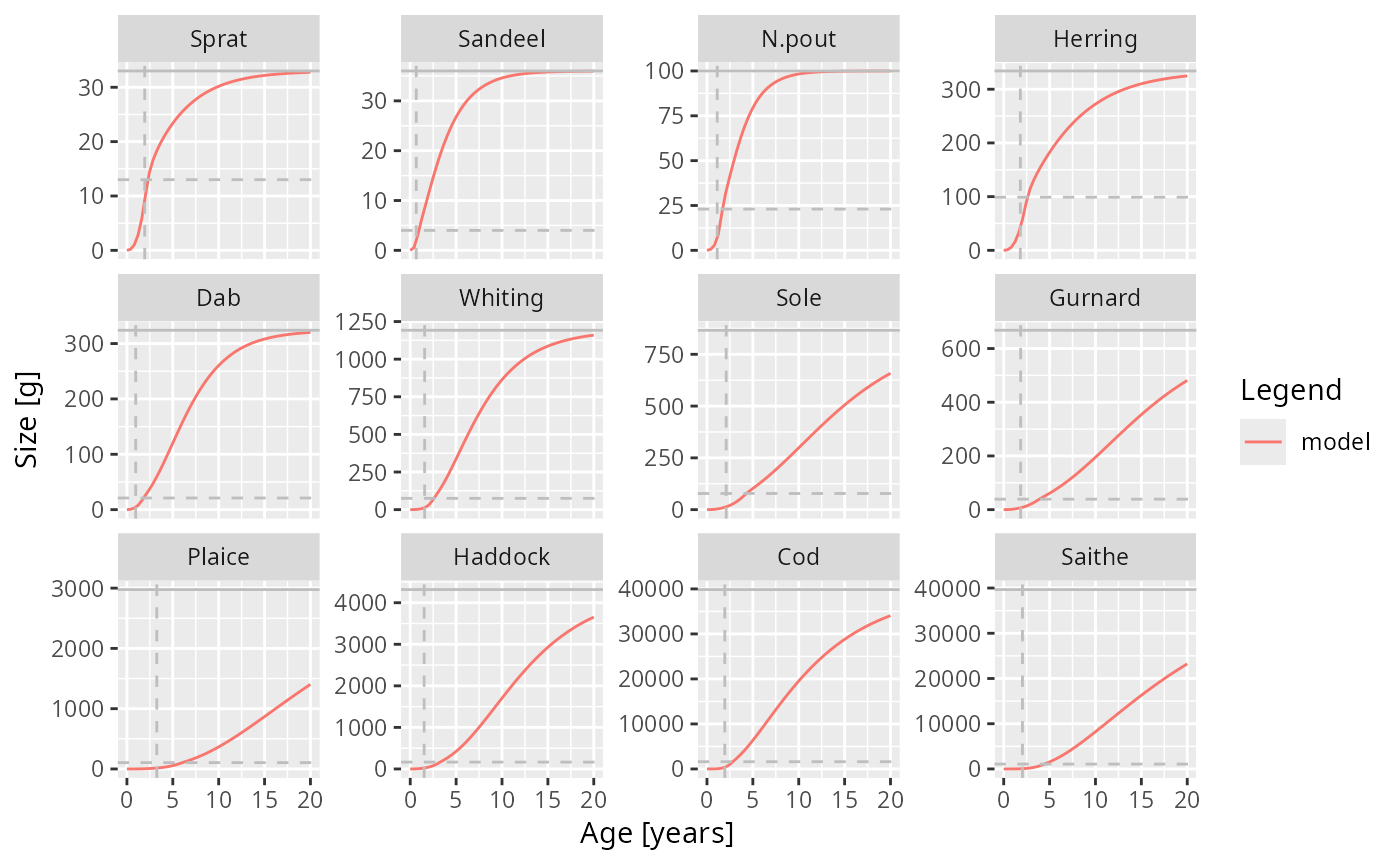When the growth curve for only a single species is plotted, horizontal lines are included that indicate the maturity size and the maximum size for that species. If furthermore the species parameters contain the variables a and b for length to weight conversion and the von Bertalanffy parameter k_vb (and optionally t0), then the von Bertalanffy growth curve is superimposed in black.

plotGrowthCurves(
object,
species = NULL,
max_age = 20,
percentage = FALSE,
species_panel = FALSE,
highlight = NULL,
return_data = FALSE,
...
)

plotlyGrowthCurves(
object,
species = NULL,
max_age = 20,
percentage = FALSE,
species_panel = FALSE,
highlight = NULL
)

## Arguments

object MizerSim or MizerParams object. If given a MizerSim object, uses the growth rates at the final time of a simulation to calculate the size at age. If given a MizerParams object, uses the initial growth rates instead. The species to be selected. Optional. By default all target species are selected. A vector of species names, or a numeric vector with the species indices, or a logical vector indicating for each species whether it is to be selected (TRUE) or not. The age up to which to run the growth curve. Default is 20. Boolean value. If TRUE, the size is given as a percentage of the maximal size.If TRUE, display all species with their Von Bertalanffy curves as facets (need species and percentage to be set to default). Default FALSE. Name or vector of names of the species to be highlighted. A boolean value that determines whether the formatted data used for the plot is returned instead of the plot itself. Default value is FALSE Other arguments (currently unused)

## Value

A ggplot2 object

plotting_functions

Other plotting functions: animateSpectra(), plot,MizerSim,missing-method, plotBiomass(), plotDiet(), plotFMort(), plotFeedingLevel(), plotPredMort(), plotSpectra(), plotYieldGear(), plotYield(), plotting_functions

## Examples

# \donttest{
params <-  NS_params
sim <- project(params, effort=1, t_max=20, t_save = 2, progress_bar = FALSE)
plotGrowthCurves(sim, percentage = TRUE)plotGrowthCurves(sim, species = "Cod", max_age = 24)plotGrowthCurves(sim, species_panel = TRUE)# Returning the data frame
fr <- plotGrowthCurves(sim, return_data = TRUE)
str(fr)
#> 'data.frame':	600 obs. of  4 variables:
#>  $Species: Factor w/ 12 levels "Sprat","Sandeel",..: 1 2 3 4 5 6 7 8 9 10 ... #>$ Age    : num  0 0 0 0 0 0 0 0 0 0 ...
#>  $value : num 0.001 0.001 0.001 0.001 0.001 0.001 0.001 0.001 0.001 0.001 ... #>$ Legend : chr  "model" "model" "model" "model" ...# }